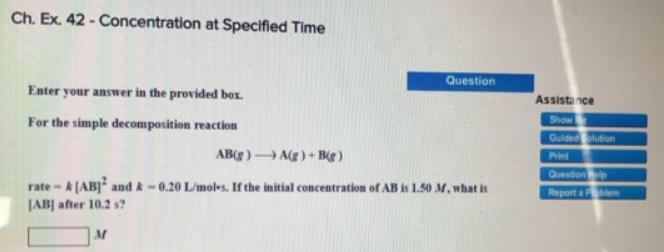# Problem: Enter your answer in the provided box. For the simple decomposition reaction AB (g) → A (g) + B (g) rate = k[AB]2 and k = 0.20 L/mol • s. If the initial concentration of AB is 1.50 M, what is [AB] after 10.2 s?

###### FREE Expert Solution###### Problem Details

For the simple decomposition reaction

AB (g) → A (g) + B (g)

rate = k[AB]2 and k = 0.20 L/mol • s. If the initial concentration of AB is 1.50 M, what is [AB] after 10.2 s?